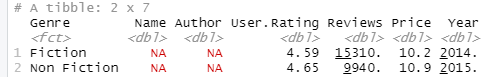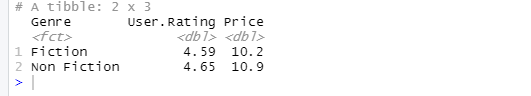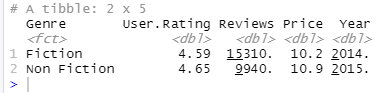GeeksforGeeks App
Open AppBrowser
Continue

# Get the summary of dataset in R using Dply

In this article, we will discuss how to get a summary of the dataset in the R programming language using Dplyr package. To get the summary of a dataset summarize() function of this module is used. This function basically gives the summary based on some required action for a group or ungrouped data, which in turn helps summarize the dataset.

Syntax: summarize(action)

The dataset in use: bestsellers3

Here, action can be any operation to be performed on grouped data, it can be frequency count, mean, average, etc.

Example: Summarize the dataset using summarize()

## R

 `library``(dplyr)`` ` `data<-``read.csv``(``"bestsellers.csv"``)``data %>% ``group_by``(Genre) %>%``  ``summarize``(``n``())`

Output:

```# A tibble: 2 x 2
Genre       `n()`
<fct>       <int>
1 Fiction        82
2 Non Fiction   117```

## Summarize ungrouped dataset

It is also possible to summarize ungrouped data. There are three possible functions that can be used for this.

• summarize_all().
• summarize_at().
• summarize_if().

### summarize_all():

summarize_all() function summarizes all the columns based on the action to be performed.

Syntax: summarize_all(action)

## R

 `library``(dplyr)`` ` `data<-``read.csv``(``"bestsellers.csv"``)``data %>% ``group_by``(Genre) %>%``  ``summarize_all``(mean)`

Output:### summarize_at():

summarize_at() function is used to apply a required action to some specific columns and generate a summary based on that

Syntax: summarize_at(vector_of_columns,action)

## R

 `library``(dplyr)`` ` `data<-``read.csv``(``"bestsellers.csv"``)``data %>% ``group_by``(Genre) %>%``  ``summarize_at``(``c``(``'User.Rating'``,``'Price'``),mean)`

Output:### summarize_if():

summarize_if() function is used to get dataset summary if a certain condition is specified.

Syntax: summarize_if(condition, action)

## R

 `library``(dplyr)`` ` `data<-``read.csv``(``"bestsellers.csv"``)``data %>% ``group_by``(Genre) %>%``  ``summarize_if``(is.numeric, mean)`

Output:My Personal Notes arrow_drop_up